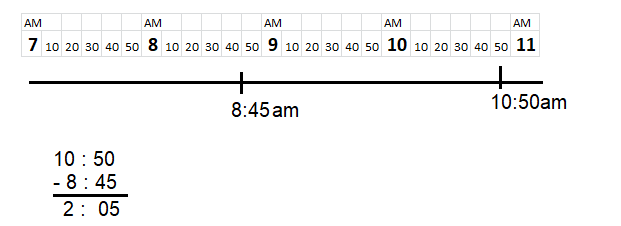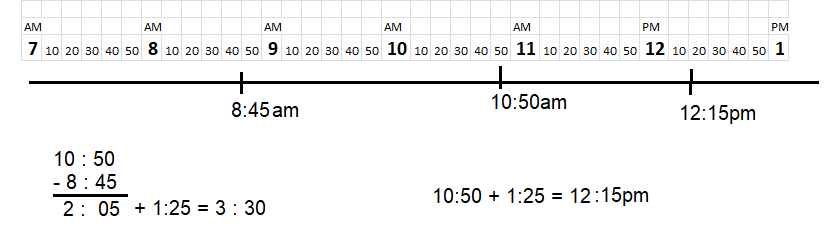# Math in Focus Grade 3 Cumulative Review Chapters 16 to 19 Answer Key

This handy Math in Focus Grade 3 Workbook Answer Key Cumulative Review Chapters 16 to 19 detailed solutions for the textbook questions.

## Math in Focus Grade 3 Cumulative Review Chapters 16 to 19 Answer Key

Fill in the blanks with past or to. (Lesson 16.1)

Question 1.
12 minutes __________ 8 is 8:12.
12 minutes past 8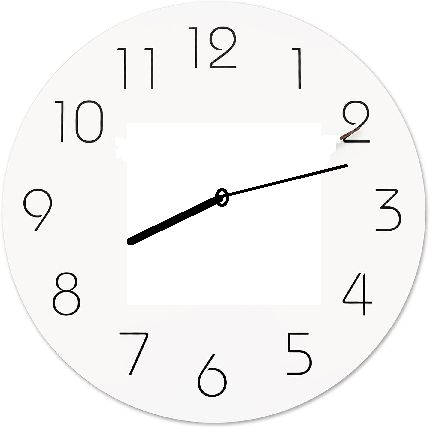Question 2.
10 minutes ___________ 3 is 2:50.
10 minutes to 3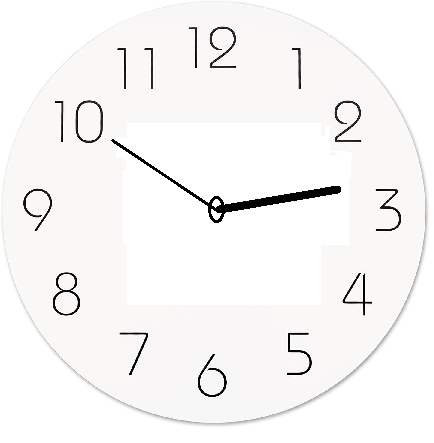Fill in the blanks. (Lesson 16.2)

Question 3.
4 h 25 min = ___________ min
Explanation: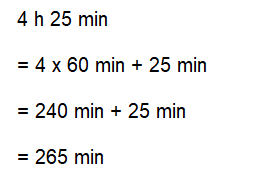Question 4.
210 min = ___________ h __________ min
Explanation: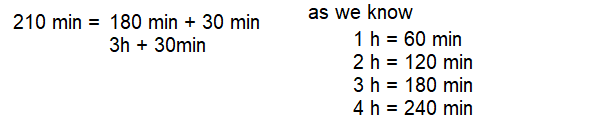Circle the correct number. (Lesson 16.6)

Question 5.
Jamie is playing basketball. The temperature outside is about (32, 72, 120) °F.
Explanation:
In lesson 6.6 the temperature scale is given,
by using the given data we can solve.
The suitable temperature to play outside is about ( 72) °F

Question 6.
Water freezes at (0, 10, 32) °F.
Explanation:
Fahrenheit temperature scale, scale based on 32° for the freezing point of water
and 212° for the boiling point of water,
the interval between the two being divided into 180 equal parts.

Question 7.
Gary puts on his jacket before he leaves the house. The temperature outside is about (50, 72, 105) °F.
Explanation:
The temperature outside is about (50) °F.
Gary puts on his jacket before he leaves the house.

Complete. Look at the angle. (Lesson 17.1)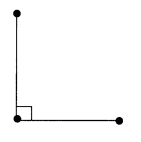Question 8.
Circle the angles below that are greater than the given angle.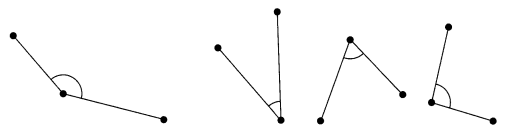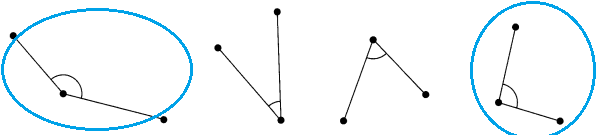Explanation:
Given angle is 90° Right angle.
The angles first and last are below that are greater than the given angle.

Question 9.
Name the pairs of perpendicular and parallel line segments.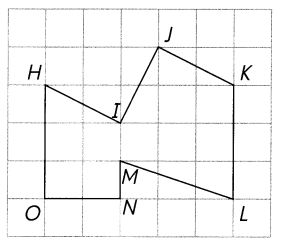Perpendicular line segments: (Lesson 17.4)
_______________________
Parallel line segments: (Lesson 17.6)
_______________________
Perpendicular line segments
HO perpendicular to ON
HI perpendicular to IJ
MN perpendicular to ON

Parallel line segments
HO and KL are Parallel line segments
HI  and  JK are Parallel line segments
Explanation:
Perpendicular line segments are line segments that intersect and make 90 degree angles.
Parallel line segments are always the same distance apart, they will never meet.

Question 10.
Mark the right angles in each figure. (Lesson 17.3)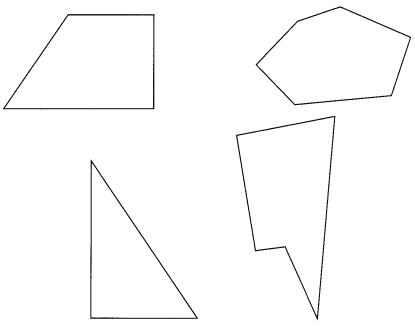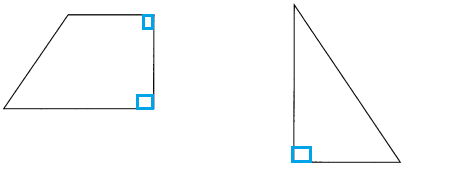Explanation:
When two straight lines intersect each other at 90˚or perpendicular to each other at the intersection,
they form the right angle.
A right angle is represented by the symbol ∟.

Circle the polygons. (Lesson 18.1)

Question 11.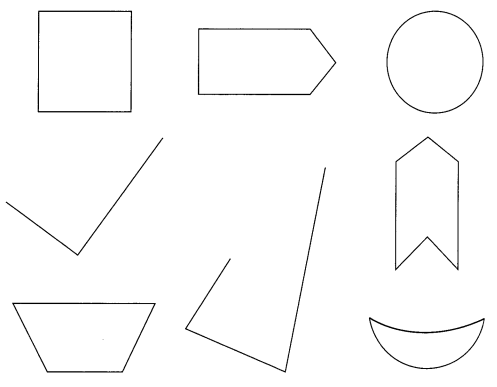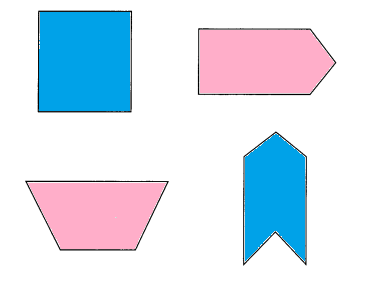Explanation:
A polygon is a closed shape with straight sides.
Rectangles, triangles, hexagons, and octagons are all examples of polygons.
It simply means many (poly) angles (gon).

Write true or false. (Lesson 18.1)

Question 12.
A trapezoid has four angles. ___________
Explanation:
A trapezoid is a quadrilateral, which means it has four sides.
Two sides must be parallel to each other for it to be a trapezoid.
A trapezoid also has four angles.

Question 13.
A circle is a quadrilateral. ____________
Explanation:
A quadrilateral is a polygon in Euclidean plane geometry with four edges (sides) and four vertices (corners).

Edges and vertices: 4

Question 14.
A rhombus has only one pair of parallel lines. ____________
False (2 pairs of parallel lines)
Explanation:
In plane Euclidean geometry, a rhombus is a quadrilateral whose four sides all have the same length.
Another name is equilateral quadrilateral, since equilateral means that all of its sides are equal in length.
A rhombus with right angles is a square.

Question 15.
An octagon has eight sides. ____________
Explanation:
An octagon is an eight-sided polygon or 8-gon.
A regular octagon has Schläfli symbol {8} and can also be constructed as a quasiregular truncated square, t{4}, which alternates two types of edges.

Question 16.
A square is not a rectangle. ____________
Explanation:
A square is a figure with four equal sides and four right angles.
It’s a popular shape for windows and record albums, among many other things.

Does the figure show a flip? Write yes or no. (Lesson 18.2)

Question 17.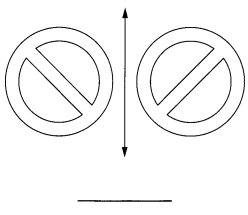Explanation:
A flip is also called a reflection.
You can see the result of a flip or a reflection in a shape that has line symmetry,
so line symmetry is related to the movement that is a reflection or a flip.

Question 18.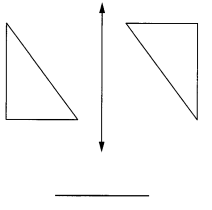Explanation:
The above image does not show flip.
We cannot draw line symmetry and it is not a reflection of image.

Does the figure show a flip? Write yes or no. (Lesson 18.2)

Question 19.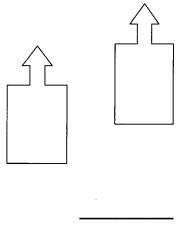Explanation:
A flip is also called a reflection.
You can see the result of a flip or a reflection in a shape that has line symmetry,
so line symmetry is related to the movement that is a reflection or a flip.

Question 20.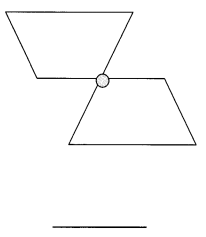Explanation:
A flip is also called a reflection.
You can see the result of a flip or a reflection in a shape that has line symmetry,
so line symmetry is related to the movement that is a reflection or a flip.

Circle the congruent shapes. (Lesson 18.2)

Question 21.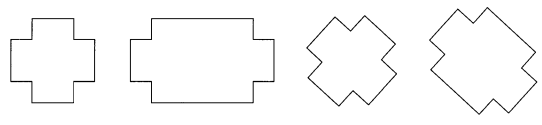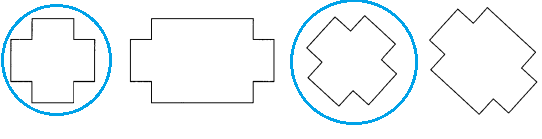Explanation:
If two figures or objects have the same shape and size or known as congruent,
or if one has the same shape and size as the mirror image of the other.

Decide if each figure is symmetric. Write yes or no. (Lesson 18.3)

Question 22.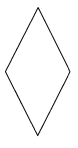Explanation:
Symmetry defines that one shape is exactly like the other shape when it is moved, rotated, flipped.
Which can exactly divide into two equal halves.

Question 23.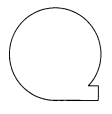Explanation:
The given image is not symmetry.
We can’t divide into two equal halves.

Question 24.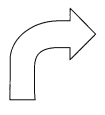Explanation:
The given image is not symmetry.
We can’t divide into two equal halves.

Decide if each figure show a line of symmetry. Write yes or no.

Question 25.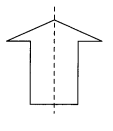Explanation:
The line of symmetry is the imaginary line that divides an object into two identical halves,
either horizontally or vertically.

Question 26.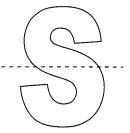Explanation:
The line of symmetry is the imaginary line that divides an object into two identical halves,
either horizontally or vertically.

Question 27.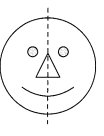Explanation:
The line of symmetry is the imaginary line that divides an object into two identical halves,
either horizontally or vertically.

Find the area of each figure. (Lesson 19.2)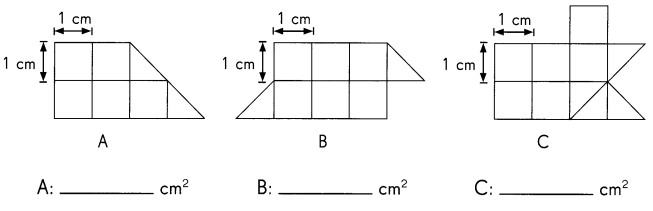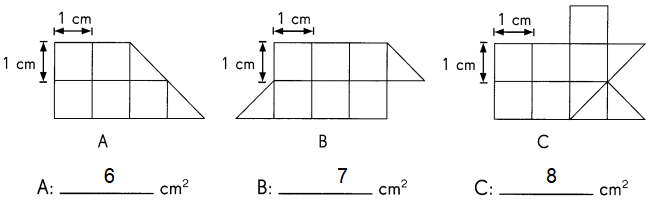Explanation:
In the above picture each area of the square is measured as 1 square centimeter.
In figure A there are 5 full square units and 2 half square units.
In figure B there are 6 full square units and 2 half square units.
In figure C there are 6 full square units and 4 half square units.

Question 28.
Which figure has the greatest area? Figure ____________
Figure C
Explanation:
By comparing the above given figures,
figure C has the greatest area.
Question 29.
Which figure has the least area? Figure ____________
Figure A
Explanation:
By comparing the above given figures,
figure A has the least area.

Complete. (Lesson 19.5)

Question 30.
Measure in inches.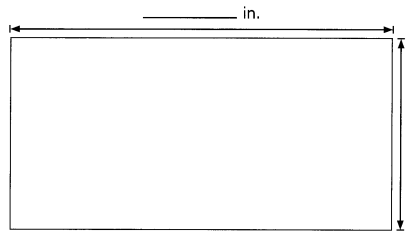__________ in.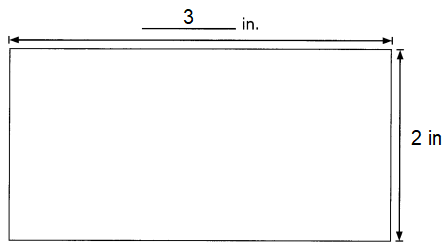Explanation:
The Area of a rectangle is the total distance covered by its boundaries or the sides.
Since there are four sides of a rectangle,
Area of the rectangle length x width.
A = 3 + 2 = 5 cm

Question 31.
The perimeter of the rectangle is _____________
Explanation:
The perimeter of a rectangle is the total distance covered by its boundaries or the sides.
Since there are four sides of a rectangle,
thus, the perimeter of the rectangle will be the sum of all four.
P = 3 + 2 + 3 + 2 = 10 in.

Problem Solving

Complete. Draw two different rectangles with a perimeter of 10 feet each.

Question 32.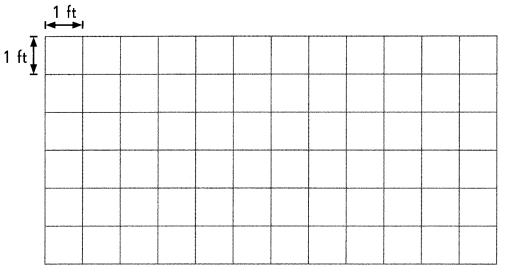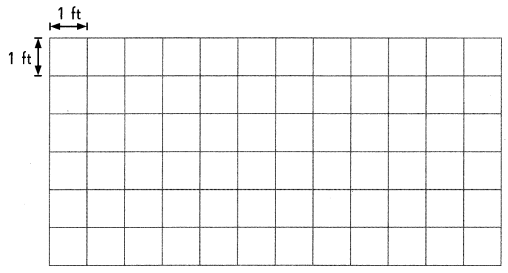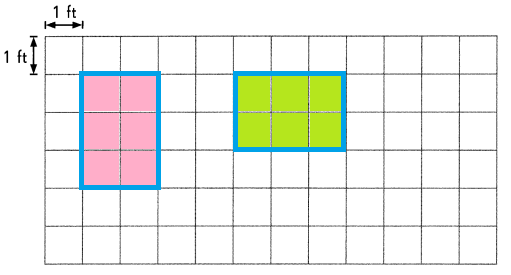Explanation:
Perimeter is the distance around the edge of a shape.
Area is used to define the amount of space taken up by a 2D shape or surface.
We measure area in square units : cm² or m².
Area is calculated by multiplying the length of a shape by its width.
Question 33.
Are the areas of the two rectangles the same? ___________
Explanation:
Both the rectangles have the same number of square units.

Question 34.
If their areas are the same, what is the area? _____________
6 Square feet
Explanation:
Each square unit represent 1 square unit.
So, there are 6 units in each rectangle.

Question 35.
If their areas are not the same, what is the difference in their areas?
Might be the difference is there size and shape.
Explanation:
If we take different number of square boxes in both the rectangles,
then we find the difference in their areas.

Complete.

Question 36.
Look at the shape in a.
How many sides and angles are there?
6 sides are there.
Explanation:
It represents hexagon.
A hexagon can be defined as polygon with six sides.
The two-dimensional shape has 6 sides, 6 vertices and 6 angles.

Question 37.
Draw another shape that has the same number of sides and angles.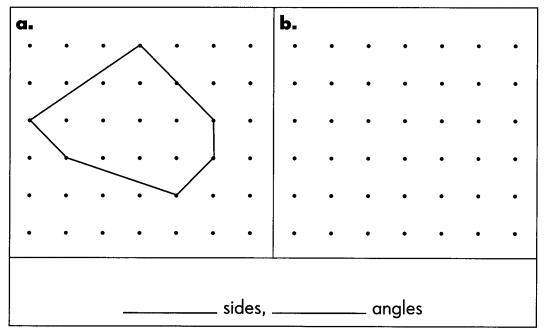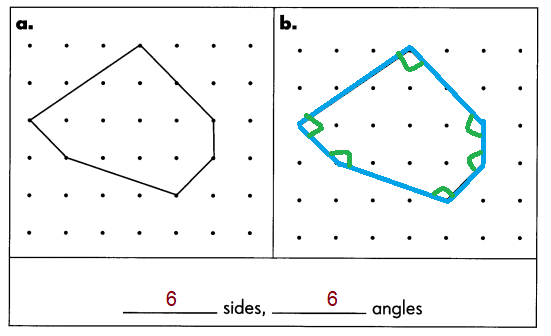Explanation:
It represents hexagon.
A hexagon can be defined as polygon with six sides.
The two-dimensional shape has 6 sides, 6 vertices and 6 angles.

Question 38.
On Monday, Samantha spent 8 hours 10 minutes in school. On Tuesday, she spent 6 hours 55 minutes in school. How much longer did she spend in school on Monday than on Tuesday?
1h: 15 min
Explanation:
8 : 10 –  6 : 55
6 : 55 is subtracted from 8 : 10 min time
7 : 60 – 6 : 55
= 1 : 10 + 5
= 1:15min
On Monday, Samantha spent 8 hours 10 minutes in school.
On Tuesday, she spent 6 hours 55 minutes in school.
Total time she spent in school on Monday than on Tuesday

Question 39.
Laura sews six square patches to make a quilt, as shown below. The side of each square patch is 15 inches long. What is the perimeter of the quilt?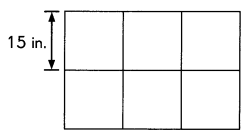Explanation:
Perimeter of a rectangle = P
P = 2l + 2w
P = 2 x 45 + 2 x 30 = 90 + 60 = 150 square inches.

Question 40.
Mr. Jones takes 2 hours 30 minutes to drive from San Diego to Los Angeles. He reaches Los Angeles at 4:25 p.m. What time did he leave San Diego?
Explanation:
Mr. Jones takes 2 hours 30 minutes to drive from San Diego to Los Angeles.
He reaches Los Angeles at 4:25 p.m.
Total time did he leave San Diego
4:25pm – 2 :30pm  = 1:55 pm

Question 41.
Rhonda read a book from 8:45 A.M. to 10:50 A.M.
a. How long did she spend reading the book?